# Broyden method

(diff) ← Older revision | Latest revision (diff) | Newer revision → (diff)

An iterative algorithm for solving non-linear equations. The equation to be solved is(a1)

where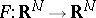is Lipschitz continuously differentiable (cf. also Lipschitz condition). Letbe the Jacobian of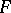.

Here, the setting is such that linear equations can be solved by direct methods and hence it is assumed that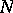is not very large or that special structure is present that permits efficient sparse matrix factorizations. Having said that, however, note that Broyden's method can be effective on certain very large problems [a14] having dense and very large Jacobians.

## Convergence properties.

The Newton method updates a current approximation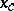to a solutionby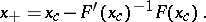(a2)

When a method is specified in terms of the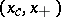-notation, it is understood that the iteration follows the rule with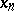playing the role ofandthat of. The classic local convergence theory for Newton's method, [a4], [a13], [a7] states that if the initial iterate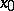is sufficiently nearandis non-singular, then the Newton iteration converges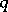-quadratically to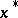. This means that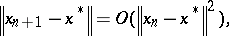(a3)

hence the number of significant figures roughly doubles with each iteration.

The cost of implementation in Newton's method is both in evaluations of functions and Jacobians and in the matrix factorization required to solve the equation for the Newton step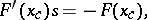(a4)

which is an implicit part of (a2). One way to reduce the cost of forming and factoring a Jacobian is to do this only for the initial iterate and amortize the cost over the entire iteration. The resulting method is called the chord method:(a5)

The chord method will converge rapidly, although not as rapidly as Newton's method, if the initial iterate is sufficiently nearand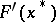is non-singular. The chord iterates satisfy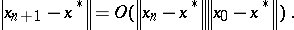(a6)

The convergence implied by (a6) is fast ifis a very good approximation to, and in such a case the chord method is recommended. The chord iteration can be quite slow or diverge completely even in cases whereis accurate enough for Newton's method to perform well and converge-quadratically.

Quasi-Newton methods (cf. also Quasi-Newton method) update both an approximation toand one to. The simplest of these is Broyden's method, [a1]. Ifand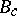are the current approximations to theand, then, similarly to Newton's method and the chord method,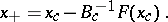(a7)

The approximate Jacobian is updated with a rank-one transformation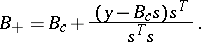(a8)

In (a8),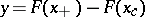and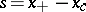.

In the case of a scalar equation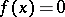,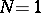and Broyden's method is the well-known secant method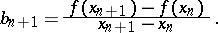The convergence behaviour, [a3], [a2], lies in between (a3) and (a6). Ifand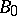are sufficiently nearandandis non-singular, then eitherfor some finiteor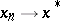-superlinearly:(a9)

Ifis singular, Newton's method and Broyden's method (but not the chord method) will still converge at an acceptable rate in many circumstances, [a10], [a11], [a12].

## Implementation.

The simple implementation from [a6], described below, is based directly on an approximation of the inverse of the Jacobian. The approach is based on a simple formula, [a8], [a9]. Ifis a non-singular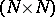-matrix and, then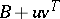is invertible if and only if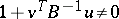. In this case(a10)

The formula (a10) is called the Sherman–Morrison formula.

To start with, note that it can be assumed that. The reason for this is that ifis a good approximation to, then one may equally well apply Broyden's method to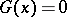with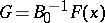and use the identity matrix as an approximation to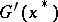. One way to do this is to form and factorand replaceby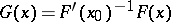. In this way, just like the chord method, the computation and factorization of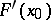is amortized over the entire iteration, but one also gets the faster convergence and enhanced robustness of Broyden's method.

In the context of a sequence of Broyden updates, forone has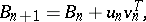where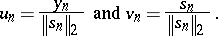Settingone sees that(a11)

Since the empty matrix product is the identity, (a11) is valid for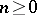.

Hence the action of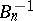on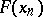(i.e., the computation of the Broyden step) can be computed from the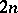vectors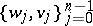at a cost offloating point operations. Moreover, the Broyden step for the following iteration is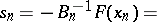(a12)Since the product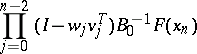must also be computed as part of the computation of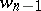, one can combine the computation ofandas follows: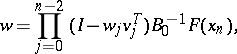(a13)The major weakness in this formulation is the need to store two new vectors with each non-linear iteration. This can be reduced to one, [a5], [a13], at the cost of a bit more complexity. This makes Broyden's method a good algorithm for very large problems if the product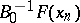can be evaluated efficiently.

A completely different approach, [a4], is to perform a QR-factorization (cf. Matrix factorization; Triangular matrix) ofand update the QR-factors. This is more costly than the approach proposed above, requiring the storage of a full-matrix and an upper triangular-matrix and more floating point arithmetic. However, this dense matrix approach has better theoretical stability properties, which may be important if an extremely large number of non-linear iterations will be needed.

How to Cite This Entry:
Broyden method. Encyclopedia of Mathematics. URL: http://encyclopediaofmath.org/index.php?title=Broyden_method&oldid=16772
This article was adapted from an original article by C.T. Kelley (originator), which appeared in Encyclopedia of Mathematics - ISBN 1402006098. See original article